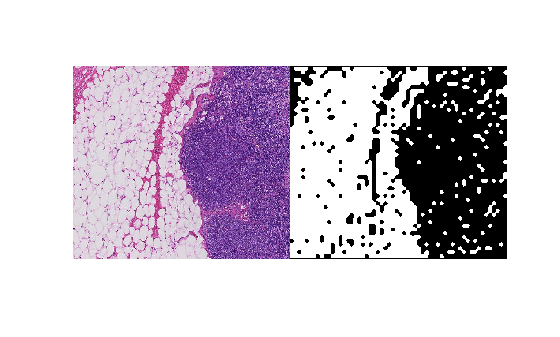# world2sub

Convert world coordinates to pixel subscripts

## Syntax

``pixelsub = world2sub(bim,world)``
``pixelsub = world2sub(bim,world,'Level',L)``

## Description

example

````pixelsub = world2sub(bim,world)` converts the world coordinates, `world`, to the corresponding pixel subscripts, `pixelsub`. The world coordinates should be in the same order as the pixel subscripts.```
````pixelsub = world2sub(bim,world,'Level',L)` additionally specifies the resolution level to use in a multi-resolution image. By default, `Level` is `1`.```

## Examples

collapse all

Convert pixel subscripts from one level to another via the world coordinates to refer to the same spatial region.

Create a blocked image from a sample image included with the toolbox.

`bim = blockedImage('tumor_091R.tif');`

Define a region of interest in the finest resolution level in pixel subscripts.

```level1PixelSubStart = [1700, 1550 1]; level1PixelSubEnd = [2100, 2000 3];```

Get the image data from the region of interest on the resolution level 1 image.

```imr = getRegion(bim, level1PixelSubStart, level1PixelSubEnd, "Level", 1); size(imr)```
```ans = 1×3 401 451 3 ```

Convert the pixel subscripts that define the region of interest into world coordinates. By default, `sub2world` converts the coordinates at level 1, the finest resolution.

`worldRegion = sub2world(bim,[level1PixelSubStart; level1PixelSubEnd]);`

Compute a binary mask at the coarsest level.

`bbw = apply(bim, @(bs)imbinarize(im2gray(bs.Data)), "Level", 3);`

Convert the world coordinates of the region of interest to pixel subscripts of the mask. Note that the mask has only two dimensions.

```worldRegion = worldRegion(:,1:2); maskPixelSubs = world2sub(bbw,worldRegion);```

```bwr = getRegion(bbw, maskPixelSubs(1,:), maskPixelSubs(2,:)); size(bwr)```
```ans = 1×2 51 58 ```

View the original image and the mask.

`montage({imr,bwr})`## Input Arguments

collapse all

Blocked image, specified as a `blockedImage` object.

World coordinates, specified as a K-by-N numeric matrix, where K is the number of world coordinate vectors and N is the number of dimensions of the `blockedImage`.

Data Types: `single` | `double` | `int8` | `int16` | `int32` | `int64` | `uint8` | `uint16` | `uint32` | `uint64` | `logical`

## Output Arguments

collapse all

Pixel subscripts, returned as a K-by-N integer-valued matrix, where N is the number of dimensions and K is the number of coordinates.

## Tips

• World coordinates are theoretically continuous domain values represented by floating point numbers. Subscripts are discrete integer values that can be used to index into the underlying array. Floating point computation and rounding may cause small changes in world coordinates around the edge of pixels to map to different neighboring subscript locations. `world2sub` rounds up world coordinate values on the edge of two pixels, except for pixels on the border, where it rounds down to the last pixel.

## Version History

Introduced in R2021a